• Shuffle
Toggle On
Toggle Off
• Alphabetize
Toggle On
Toggle Off
• Front First
Toggle On
Toggle Off
• Both Sides
Toggle On
Toggle Off
Toggle On
Toggle Off
Front

## Card Range To Study

throughPlay buttonPlay buttonProgress

1/24

Click to flip

Use LEFT and RIGHT arrow keys to navigate between flashcards;

Use UP and DOWN arrow keys to flip the card;

H to show hint;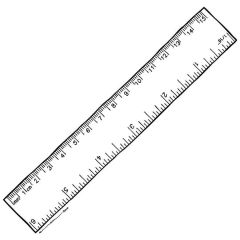1.1: Length and Time Ruler: Used to measure lengths for distances between 1mm and 1m.To find the volume of a regular object, you can use a mathematical formula, you just need to make a couple of length measurements. Measuring cylinders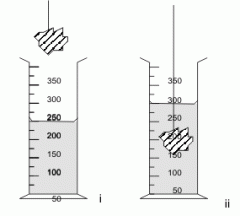To measure the volume of anirregular object, you have to put the object into measuring cylinder with water. When you add the object, it displaces the water, making the water level rise. Measure this rise to give the volume of the object. Micrometers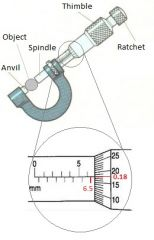TimeAn interval of time is measured using clocks.To find the amount of time it takes a pendulum to make a spin, time ~25 circles and then divide by same number as the number of circles.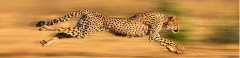1.2: Motion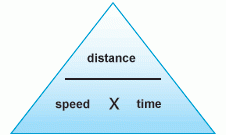Average speed equation: total distance/total timeAcceleration: change in velocity/time taken Definitions Speed: Distance travelled per unit time.Velocity: The rate of increase of distance travelled in a specifieddirection with time. Speed/Time Graph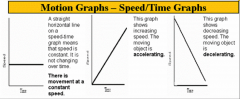A straight horizontal line means that speed is constant.If the line is increasing, the moving object is accelerating.If the line is decreasing, it means the moving object is decelerating. Distance/Time Graph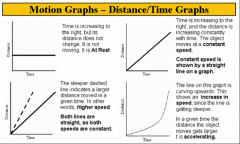Time is increasing to the right, but its distance does not change, so it is at rest.The time and distance is increasing constantly, so it moves at a constant speed. Summary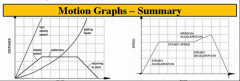Acceleration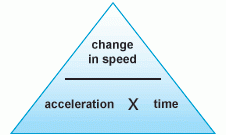An increase in speed is a positive acceleration.A decrease in speed is a negative acceleration/ deceleration.If the acceleration isn't constant, the line on the graph will be curved. Acceleration and Gravity The downward acceleration of an object is caused by gravity. This happens most when an object is in free fall.Objects are slowed down by air resistance. Once the air resistance is equal to the force of gravity, the object has reached terminal velocity. This means that it will stay at a constant velocity. GravityThe value of gravity on Earth is 9.81m/s2. However, 10m/s2 is used in most calculations.Gravity= 2 x height dropped/ (time)2g=2h/t2This only works when there is no air resistance, so a vacuum chamber is required.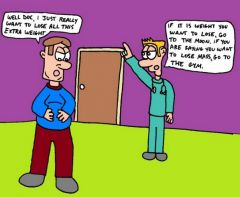1.3: Mass and Weight Mass: The property of an object that is a measure of its resistance to accelerate, the amount of matter it contains and its influence in a gravitational field.Weight: It is the force of gravity acting on an object, measured in Newtons, and given by this formula:Weight= mass x acceleration due to gravity.Weights may be compared using a balance.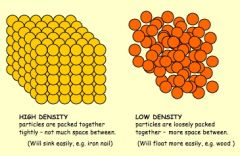1.4: Density To determine the density of a liquid place a measuring cylinder with some liquid. The change in mass is the mass of the liquid and the volume is shown on the sale, then use this formula:Density= mass/volumeTo determine the density of an object, you use the methods used in 1.1 to find the volume and then weigh the object and use the formula.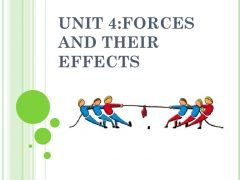1.5: Forces (i) Effects of forces A force may produce a change in size and shape of a body, give theacceleration or deceleration or a change in direction depending on the direction of the force. Extension/Load Graph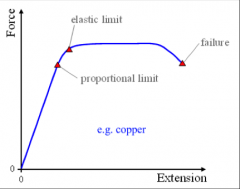Experiment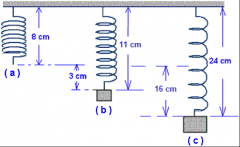Finding the resultant force of two or more forces acting along the same line: Experiment Continued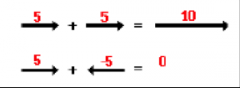Hooke's Law Hooke's law: Springs extend in proportion to load, as long as they are under their proportional limit.Equation: Load (N) = spring constant (N/mm) x extension (mm)F=kx Definitions Limit of proportionality: Point at which load and extension are no longer proportional.Elastic limit: Point at which the spring will not return to its original shape after being stretched.Force = mass x acceleration Newtons Forces are measured in Newtons. 1 Newton is the amount of force needed to give 1kg an acceleration of 1m/s2 (if you think about it using the equation it's really obvious: if force = mass x acceleration, then 1 Newton = 1kg x 1m/s2) Circular Motions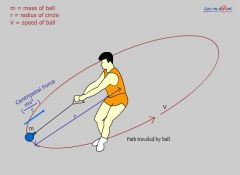An object at a steady speed in a circular orbit is always accelerating as its direction is changing, but it gets no closer to the centre. Centripetal force This is the force acting towards the centre of a circle.It is a force that is needed (not caused by) a circular motion, e.g.: when you swing a ball on a string round a circle, the tension of the string is the centripetal force. If the string is cut, then the ball will travel in a straight line at a tangent to the circle at the point where the string was cut (Newton's first law). Centrifugal force This is also know as the non-existent force is the force acting away the centre of a circle. This is what makes a slingshot go outwards as you spin it. The centrifugal force is the reaction to the centrifugal force (Newton's third law). It has the same magnitude, but opposite direction to the centrifugal force ("equal but opposite").centripetal force = mass x velocity2 / radius.